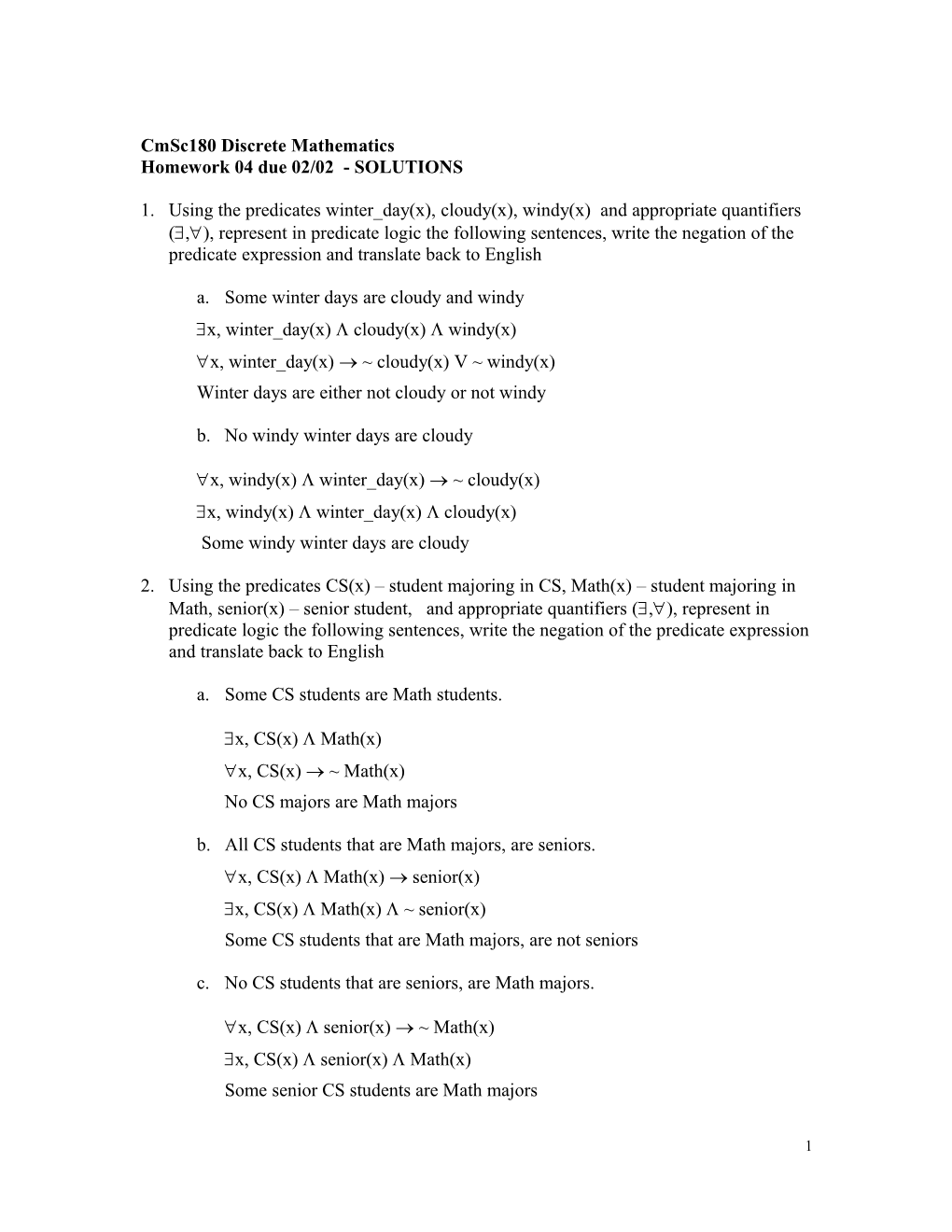# Cmsc180 Discrete MathematicsCmSc180 Discrete Mathematics

Homework 04 due 02/02 - SOLUTIONS

1. Using the predicates winter_day(x), cloudy(x), windy(x) and appropriate quantifiers (,), represent in predicate logic the following sentences, write the negation of the predicate expression and translate back to English
1. Some winter days are cloudy and windy

x, winter_day(x)  cloudy(x)windy(x)

x, winter_day(x)  ~cloudy(x) V ~ windy(x)

Winter days are either not cloudy or not windy

1. No windy winter days are cloudy

x, windy(x)  winter_day(x)  ~cloudy(x)

x, windy(x)  winter_day(x) cloudy(x)

Some windy winter days are cloudy

1. Using the predicates CS(x) – student majoring in CS, Math(x) – student majoring in Math, senior(x) – senior student, and appropriate quantifiers (,), represent in predicate logic the following sentences, write the negation of the predicate expression and translate back to English
1. Some CS students are Math students.

x, CS(x)  Math(x)

x, CS(x)  ~Math(x)

No CS majors are Math majors

1. All CS students that are Math majors, are seniors.

x,CS(x)  Math(x) senior(x)

x,CS(x)  Math(x)  ~senior(x)

Some CS students that are Math majors, are not seniors

1. No CS students that are seniors, are Math majors.

x,CS(x) senior(x) ~ Math(x)

x,CS(x)  senior(x)  Math(x)

Somesenior CS students are Math majors

1. No CS students are seniors and Math majors.

x,CS(x) ~(senior(x)  Math(x))

x,CS(x)  senior(x)  Math(x)

Some CS students that Math majors and seniors

1. All seniors are CS students or Math majors

x,senior(x) ( CS(x) V Math(x))

x,senior(x)  ~ (CS(x) V Math(x))

Some senior students are neither CS students nor Math students

1. Using the predicates student(x), study(x), play_soccer(x), healthy (x) and appropriate quantifiers (,), represent in predicate logic the following sentences, write the negation of the predicate expression and translate back to English
1. All students play soccer.

 x, student(x)  play_soccer(x)

x, student(x)  ~ play_soccer(x)

Some students don’t play soccer

1. No students play soccer and study

 x, student(x) ~( play_soccer(x) study(x))

x, student(x)  play_soccer(x) study(x)

Some students play soccer and study

1. Some soccer players are students

x, play_soccer(x)  student(x)

 x, play_soccer(x) ~student(x)

No soccer players are students

1. Students that are healthy, play soccer.

x, students(x) healthy(x) soccer_player(x)

x, student(x) healthy(x) ~ soccer_player(x) .

Some students are healthy but do not play soccer

1. Some healthy students do not play soccer

x, student(x)  healthy(x) ~ soccer_player(x)

x, students(x) healthy(x) soccer_player(x)

All healthy students play soccer

1. No healthy soccer players are students

x, healthy(x)  soccer_player(x) ~ students(x)

x,healthy(x)  soccer_player(x) students(x)

Some healthy soccer players are students

1. All students that are soccer players, study

x, student(x) soccer_player(x) study(x)

x,student(x)  soccer_player(x)  ~ study(x)

Some students that are soccer players do not study

1. Some soccer players are healthy students

x, soccer_player(x) healthy(x)  student(x)

x,soccer_player(x)  ~(healthy(x) student(x))

Soccer players are not healthy students

No soccer players are healthy students

1. All students that are soccer players, are healthy

x, students(x)  soccer_player(x) healthy(x)

x,students(x) soccer_player(x) ~(healthy(x)

Some students are soccer players but are not healthy

1. Using the logical equivalences show that

(~B  (A  B))  ~A  T

(~B  (A  B))  ~A 

(~B  (~A V B))  ~A 

((~B  ~A) V (~B  B))  ~A 

((~B  ~A) V F)  ~A 

(~B  ~A)  ~A 

~(~B  ~A) V ~A 

B V A V ~A 

B V T  T

1